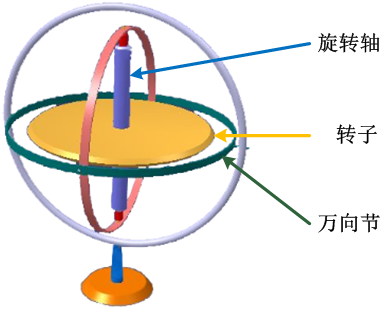# 【技术分享】九轴传感器之陀螺仪ωX = (Ax1-Ax0) / (t1 – t0)

ωY = (Ay1-Ay0) / (t1 – t0)

ωZ = (Az1-Az0) / (t1 – t0)

ωX = ((Acx/4095) * Vref  – V0ω) / Sens

ωY = ((Acy/4095) * Vref  – V0ω) / Sens

ωZ = ((Acz/4095) * Vref  – V0ω) / Sens